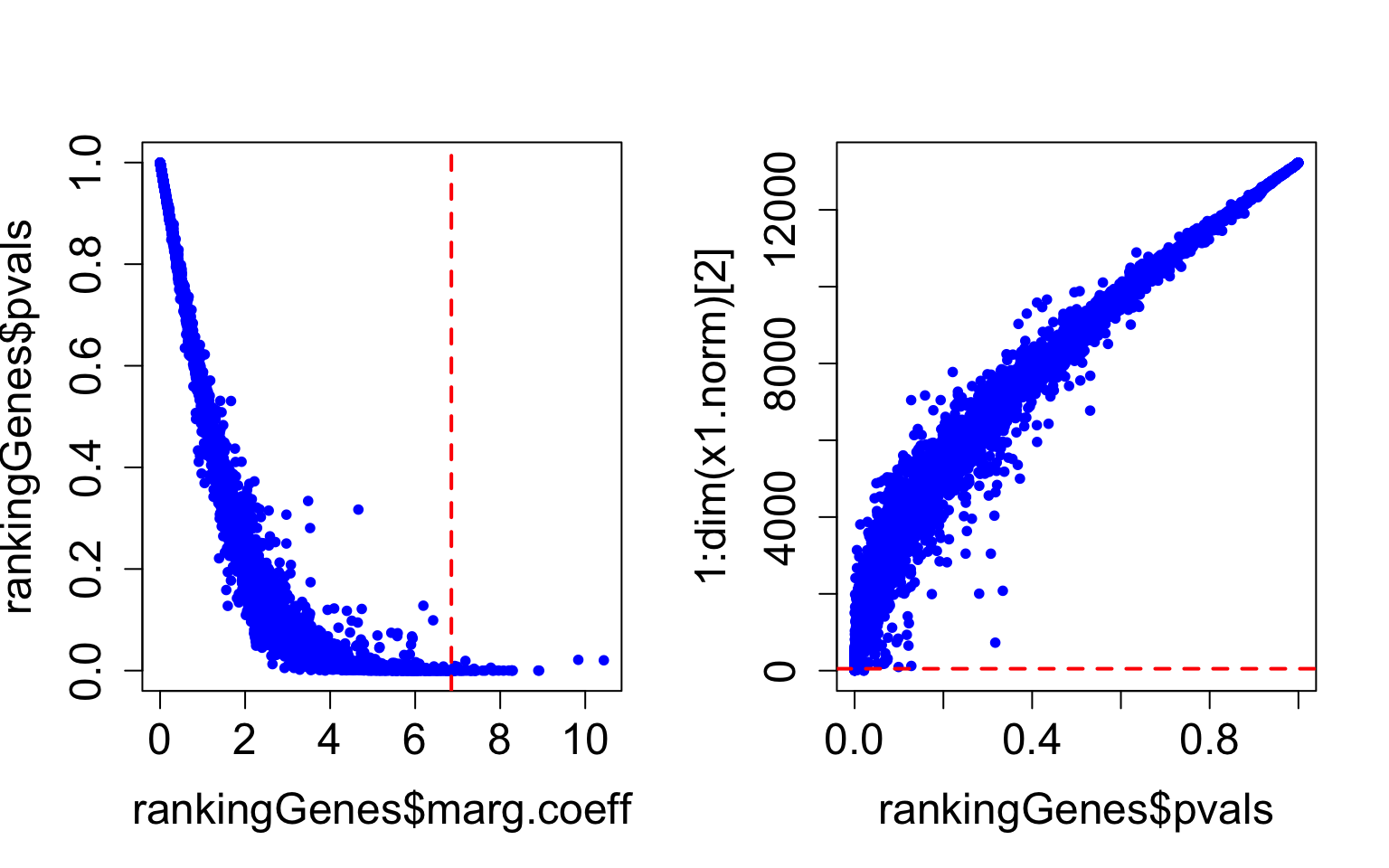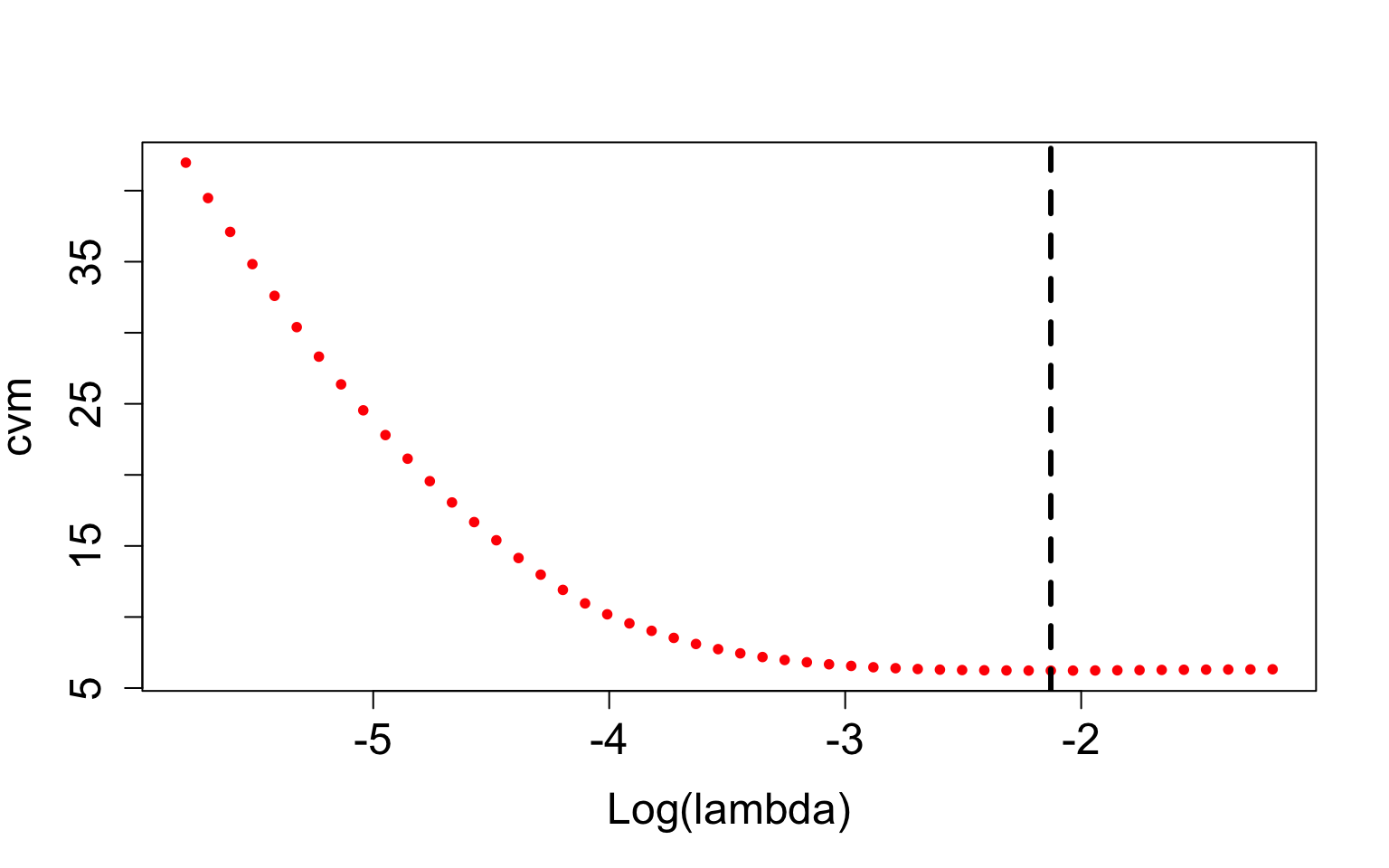# Introduction

This tutorial is a guide on how to run and use the `COSMONET R package`. It is available from http://bioinfo.na.iac.cnr.it/cosmonet/.

`COSMONET` depends on different R packages such as APML0, survival, ggpurb, igraph, pheamap.

## Installation

`COSMONET` can be installed through the following commands in the R console.

``````# ## From the command line
# ### 1. Open a terminal window
# ### 2. Go to the directory that contains your package directory.
# ### 3. Install the package
# R CMD INSTALL COSMONET_0.1.tar.gz
#
# ## From local source file
# ### Go to the link http://bioinfo.na.iac.cnr.it/cosmonet/ and download the package from the Repository - R package
# install.packages("COSMONET_0.1.0.tar.gz", repos = NULL, type="source")``````

## Using `COSMONET` to investigate cancer data

The aim of this section is to provide the users with a general view of the package, including the main functions, and to describe the package’s outputs. First, we upload the `COSMONET` R package.

``library(COSMONET)``

For illustration purposes, we use two examples of cancer data that are included in our package. The data processed by `COSMONET` are microarray from GEO database and RNA-seq data from GDC data portal. The aim of the study is to identify the genes and pathways that play a crucial role in the severity of the disease related to the survival of cancer patients.

# Example 1

For microarray data, we consider expression measurements of two different breast cancer datasets from GEO database: * GSE2034 dataset as training set (Affymetrix Human Genome U133A Array); * GSE2990 dataset as testing set (Affymetrix Human Genome U133A Array).

`RMA` (Robust Multichip Average) function from affy R package for within arrays normalization and `normalizeBetweenArrays` function from limma R package for between arrays normalization are used for the normalization of each single dataset. The annotation is made by using biomart R package after removing redundant probe sets, i.e. probe sets that measure different regions of the same gene. Generally, the pre-processing of the data are done by the users. The summary data is showed in the table below:

Summary of the breast data used for our analysis
GEO Accession Platform Nr. of genes Nr. of samples Survival data
GSE2034 Affymetrix HG-U133A 13229 286 Relapse Free Survival (RFS)
GSE2990 Affymetrix HG-U133A 13229 187 Relapse Free Survival (RFS)

In this study, the survival information is relapse-free survival, i.e., the length of time after primary treatment for a cancer ends that the patient survives without any signs or symptoms of that cancer. In other words, measure the relapse-free survival means to see how well a new treatment works. Also called DFS disease-free survival, and RFS.

To investigate the datasets, we first load the data in an R session and then, we perform the normalization between the training and testing set by using `NormalizeBetweenData()` function.

``````# Load the normalized data

# Load survival information: RFS - time (months) and status (alive=0 or death=1)

## Functional Linkage Matrix and Co-Expression Matrix

To incorporate the a-priori biological information in the penalized regression model, we need to create the network matrix. In our analysis, to construct the molecular graph, we use `CreateNetworkMatrix()` function. The disease code associated with breast cancer is (DOID:1612.

``````# Load the tissue specific data
library(tictoc)

tic("Run time")
NetworkMatrix <- CreateNetworkMatrix(
x1.norm,
repositoryDisease,
diseaseID = "DOID:1612",
topGenes = 500,
tissueSpecificEdge,
output = TRUE,
message = TRUE
)``````
``````##  "Your list contains 500 genes. 500 out of 500 are used in the network."
##  "There are 43865 edges left."``````
``toc()``
``## Run time: 297.62 sec elapsed``
``````# Network matrix
Omega <- as.matrix(NetworkMatrix\$Omega)
dim(Omega)``````
``##  13229 13229``

## Variable screenings for Cox model

In this section we present the application of the three screening techniques. We use the function `ScreeningMethod()` to identify the screened genes.

1. BioMedical Driven (BMD)-screening
``````# Selection of the BMD-screened genes
cancer.genes <- NetworkMatrix\$DiseaseGenes[,2]
screenVars.BMD <- ScreeningMethod(
x1.norm,
cancer.genes,
screening="bmd",
ranking=NULL,
thresh=NULL
)``````
``##  "Number of BMD-screened genes: 437"``
``````# Ranking of genes that are highly correlated with the patient's survival
``````##   original.index  symbol marg.coeff       beta        pvals
## 1           2449  CHRNA9  10.437125 -10.437125 2.030443e-02
## 2           3012   CXCL6   9.836375  -9.836375 2.122943e-02
## 3           9444 RACGAP1   8.915556   8.915556 1.484894e-07
## 4           7834   NR2E3   8.896399  -8.896399 8.931928e-05
## 5           2494   CKAP2   8.294733   8.294733 5.502541e-07
## 6           7414    MZB1   8.282109  -8.282109 2.372354e-04``````

The ranking of genes is obtained applying `MarginalCoxRanking()` function. Users can download the file of the ranked genes directly from the website (Repository/Data/Download Data).

``````# Plots
par(mfrow = c(1, 2))
plot(rankingGenes\$marg.coeff, rankingGenes\$pvals, col = "blue", pch = 20, cex.lab=1.5, cex.axis=1.5, cex.main=1.5, cex.sub=1.5)
# Add the threshold line related to the 50th marginal coefficient (equal to 6.851106)
abline(v=rankingGenes[floor(dim(x1.norm)/log(dim(x1.norm))),3], col="red",lwd=2, lty=2)
plot(rankingGenes\$pvals, 1:dim(x1.norm), col = "blue", pch = 20, cex.lab=1.5, cex.axis=1.5, cex.main=1.5, cex.sub=1.5)
# Add the threshold line equal to 50 (i.e., the first 50 genes)
abline(h=floor(dim(x1.norm)/log(dim(x1.norm))), col="red",lwd=2, lty=2)``````Figure 1: Plots based on the \(p\)-values and marginal coefficients of the ranked cancer genes

These plots give an idea of the number of screened genes that can be involved in the analysis to give significance to the results (in the first plot the significant genes are positioned to the right of the dashed red line; in the second one the genes are are below the dashed red line). In particular, we set the threshold equal to \(\lfloor n/ log n \rfloor=50\), i.e., the 50 first genes as DAD-screened genes.

``````# Selection of the DAD-screened genes
x1.norm,
cancer.genes,
ranking=rankingGenes,
thresh=floor(dim(x1.norm)/log(dim(x1.norm)))
)``````
``##  "Number of DAD-screened genes: 50"``
1. BioMedical Driven + DAta Driven Screening (BMD+DAD-screening)
``````# Union of BMD- and DAD-screeened genes
x1.norm,
cancer.genes,
ranking=rankingGenes,
thresh=floor(dim(x1.norm)/log(dim(x1.norm)))
)``````
``##  "Number of BMD+DAD-screened genes: 549"``

We consider as resulting set the union of the BMD and DAD-screened genes.

## Network-Regularized Cox Regression

Now we are able to apply the penalized methods using the subset of screened variables in order to identify the active regression coefficients and the optimal cutoff (based on the prognostic index) on the training set. We can get reproducible results by fixing \(alpha\) (network influence) and the elements of each folds in the 5-fold cross validation. We perform the complete procedure applying `CosmonetTraining()` and `SelectOptimalCutoff()` functions.

``````# Set alpha and lambda parameters
alpha <- 0.5
# lambda <- 10^(seq(0,-2,-0.05))``````
``````# For reproducibility of the results
library(caret)
set.seed(2021)
flds <- createFolds(y1\$time, k = 5, list = TRUE, returnTrain = FALSE)
foldids <- rep(1,dim(y1))
foldids[flds\$Fold2] = 2
foldids[flds\$Fold3] = 3
foldids[flds\$Fold4] = 4
foldids[flds\$Fold5] = 5``````
``````# BMD-screening + variable selection
# pdf(file="results/Train_KM_BMD_median.pdf",width=15, height=7)
tic("Run time")
fitTraining.BMD <- CosmonetTraining(
10,
x1.norm,
y1,
screenVars.BMD,
family="Cox",
penalty="Net",
Omega=Omega,
alpha=alpha,
nlambda = 50,
foldid = foldids,
selOptLambda="min",
optCutpoint="median"
)``````Figure 2: Training Phase (BMD-screening): cross-validation plot , Kaplan-Meier curves and distribution plot